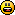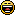###Author Topic: new QSIN (QQSIN)  (Read 15155 times)

####Quentin

• Prof. Inline
•• Posts: 915##### Re: new QSIN (QQSIN)
« Reply #15 on: 2011-Aug-08 »
like always: It depends on ....

QSIN is working faster as long as the values for the angle value are small. But often, I don't care about the value of the angle, e.g. increment per 1 at each loop for an endless rotation of a sprite. For large values for angle the native SIN funtion from GLBasic is faster.

Example:
Code: (glbasic) [Select]
`...time3 = GETTIMERALL() FOR i = 0 TO 200000 z=SIN(i) NEXTdiff3 = GETTIMERALL() - time3counter =0time4 = GETTIMERALL() FOR i = 0 TO 200000 za= QSIN (i) NEXTdiff4 = GETTIMERALL() - time4counter =0time5 = GETTIMERALL() FOR i = 0 TO 200000 zs= QSIN_OLD (i) NEXTdiff5 = GETTIMERALL() - time5...`
the bigger the values for angle, the more fast the native GLBasic SIN function is. You'll see the same effect with the precalculated SIN values (see lookup sample from Ocean)

Btw: could be interesting to look how math functions could work:

http://stackoverflow.com/questions/345085/how-do-trigonometric-functions-work/345117#345117
« Last Edit: 2011-Aug-08 by Quentin »

####Qedo##### Re: new QSIN (QQSIN)
« Reply #16 on: 2011-Aug-10 »
@ Quentin
the new QQSIN for high (and low) angle.
Ciao

####kanonet

• Prof. Inline
•• Posts: 1142##### Re: new QSIN (QQSIN)
« Reply #17 on: 2011-Aug-15 »
I tried to optimise QSIN/QQSIN and found this to be the fastest on any angle (at least on my machine):
Code: (glbasic) [Select]
`FUNCTION QQSIN: x IF x > 360 DEC x, INTEGER( x / 360 ) * 360 ELSEIF  x < 0 INC x, INTEGER( 1 - x / 360 ) * 360 ENDIF IF x < 180 x = ( 0.02222144652331750907567718514381 - 0.00012346049003081125437778164739108 * x )* x RETURN ( 0.775 + 0.225 *x ) * x ENDIF x = 180 - x x = ( 0.02222144652331750907567718514381 + 0.00012346049003081125437778164739108 * x ) * x RETURN ( 0.775 - 0.225 * x ) * xENDFUNCTION`
Lenovo Thinkpad T430u: Intel i5-3317U, 8GB DDR3, NVidia GeForce 620M, Micron RealSSD C400 @Win7 x64

####Qedo##### Re: new QSIN (QQSIN)
« Reply #18 on: 2011-Aug-15 »
Kanonet
You're right.
Your code is faster on average by 7%
well done
ciao

####Qedo##### Re: new QSIN (QQSIN)
« Reply #19 on: 2011-Aug-15 »
sorry Ocean, but I don't understand.
The angles outside 0-360 degrees are redused at the first quadrant but not converted in integer.
Can you give a practical example?

####kanonet

• Prof. Inline
•• Posts: 1142##### Re: new QSIN (QQSIN)
« Reply #20 on: 2011-Aug-15 »
Not true ocean, i think  you didnt understand the calculation.

But very interesting:

i put some DEBUGs in the code:
Code: (glbasic) [Select]
`FUNCTION QQSIN: xDEBUG "start x: "+x IF x > 360 DEC x, INTEGER( x / 360 ) * 360 ELSEIF  x < 0 INC x, INTEGER( 1 - x / 360 ) * 360 ENDIFDEBUG " calculated x: "+x IF x < 180 x = ( 0.02222144652331750907567718514381 - 0.00012346049003081125437778164739108 * x )* xDEBUG " sin(x): "+(( 0.775 + 0.225 * x ) * x)+CHR\$(10) RETURN ( 0.775 + 0.225 *x ) * x ENDIF x = 180 - x x = ( 0.02222144652331750907567718514381 + 0.00012346049003081125437778164739108 * x ) * xDEBUG " sin(x): "+(( 0.775 - 0.225 * x ) * x)+CHR\$(10) RETURN ( 0.775 - 0.225 * x ) * xENDFUNCTION`if i do:
Code: (glbasic) [Select]
`QQSIN(60.3)QQSIN(60.3+360)QQSIN(60.3-360)QQSIN(60.3+720)QQSIN(60.3-720)`i get on debugger:
Code: (glbasic) [Select]
`start x: 60.3 calculated x: 60.3 sin(x): 0.8691949954start x: 420.3 calculated x: 60.3 sin(x): 0.8691949954start x: -299.7 calculated x: 60.3 sin(x): 0.8691949954start x: 780.3 calculated x: 60.3 sin(x): 0.8691949954start x: -659.7 calculated x: 60.3 sin(x): 0.8691949954`so basically no difference.

But by coding
Code: (glbasic) [Select]
`DEBUG (QQSIN(60.3)-QQSIN(60.3+360))`i get:
Code: (glbasic) [Select]
`-1.110223025e-016`so abruptly there s a very, very small difference... but i think its so small, i think it doesnt matter, 'cuz if you need high precision, you take the GLBasic SIN() and not one of the quicker functions.
Lenovo Thinkpad T430u: Intel i5-3317U, 8GB DDR3, NVidia GeForce 620M, Micron RealSSD C400 @Win7 x64

####Qedo##### Re: new QSIN (QQSIN)
« Reply #21 on: 2011-Aug-17 »
in the Kanonet code eliminated the integer cast  (see code) Now >10% faster (on my computer)

Code: (glbasic) [Select]
`FUNCTION QQSIN1: x // KanonetLOCAL xx%xx=xIF xx >= 360 DEC x, ( xx / 360 ) * 360  // was DEC x, INTEGER( x / 360 ) * 360 ELSEIF  x < 0 INC x, ( 1 - xx / 360 ) * 360 // was INC x, INTEGER( 1 - x / 360 ) * 360ENDIF IF x < 180 x = ( 0.02222144652331750907567718514381 - 0.00012346049003081125437778164739108 * x )* x RETURN ( 0.775 + 0.225 *x ) * x ENDIF x = 180 - x x = ( 0.02222144652331750907567718514381 + 0.00012346049003081125437778164739108 * x ) * x RETURN ( 0.775 - 0.225 * x ) * xENDFUNCTION`
@Ocean
your code is very very fast (40% faster) congratulations.
Ingenious the & bAnd control in: return _cos_tbl_[ (int)(x * __d__) & __c__] ;
the other side of the coin is the increased use of memory of the lookup table.
Ciao

####ampos

• Prof. Inline
••• Posts: 1599##### Re: new QSIN (QQSIN)
« Reply #22 on: 2011-Aug-17 »
I am still amazed that there is a faster formula than just taking a value from a matrix...check my web and/or my bloghttp://diniplay.blogspot.com (devblog)
http://www.ampostata.org
http://ampostata.blogspot.com
I own PC-Win, MacBook 13", iPhone 3G/3GS/4G and iPAC-WinCE

####Qedo##### Re: new QSIN (QQSIN)
« Reply #23 on: 2011-Aug-17 »
The Ocean's code use a lookup table and take a value from a matrix.
It's the fastest.
Ciao

####kanonet

• Prof. Inline
•• Posts: 1142##### Re: new QSIN (QQSIN)
« Reply #24 on: 2011-Aug-18 »
Qedo, you forgot one float, it should be:
Code: (glbasic) [Select]
`FUNCTION QQSIN: x // by Kitty Hello, Qedo, Kanonet LOCAL xx%=x IF xx > 360 DEC x, INTEGER( xx / 360 ) * 360 ELSEIF  xx < 0 INC x, INTEGER( 1 - xx / 360 ) * 360 ENDIF IF x < 180 x = ( 0.02222144652331750907567718514381 - 0.00012346049003081125437778164739108 * x )* x RETURN ( 0.775 + 0.225 *x ) * x ENDIF x = 180 - x x = ( 0.02222144652331750907567718514381 + 0.00012346049003081125437778164739108 * x ) * x RETURN ( 0.775 - 0.225 * x ) * xENDFUNCTION`
On my machine Oceans Code only need 50%-75% of the time... but if you want to save the additional memory or dont want to use INLINE, i think our code is good too.
Lenovo Thinkpad T430u: Intel i5-3317U, 8GB DDR3, NVidia GeForce 620M, Micron RealSSD C400 @Win7 x64

####Qedo##### Re: new QSIN (QQSIN)
« Reply #25 on: 2011-Aug-18 »
Kanonet, the float var controls the negative angles between -1 and 0.
The  integer xx truncated  to 0.
Also the two INTEGER() cast don't need because the whole operations are with integer variables

I like "// by Kitty Hello, Qedo, Kanonet"ciao

Code: (glbasic) [Select]
`Qedo, you forgot one float, it should be:Code: [Select]FUNCTION QQSIN: x // by Kitty Hello, Qedo, Kanonet LOCAL xx%=x IF xx > 360 DEC x, INTEGER( xx / 360 ) * 360 ELSEIF  xx < 0 INC x, INTEGER( 1 - xx / 360 ) * 360 ENDIF`

####kanonet

• Prof. Inline
•• Posts: 1142##### Re: new QSIN (QQSIN)
« Reply #26 on: 2011-Aug-18 »
@Ocean:
thanks for your explanation on platform compatibility, good to know, that it will work on every one. Btw. can users of the free version use INLINE?

@Qedo:
i dont think, that that truncating -0.5 to 0 is a problem, we have still 'good' precision. But what do you mean with "Also the two INTEGER() cast don't need because the whole operations are with integer variables"? We need INTEGER() because its part of a modulo operation. Do you want to change something on that code, or is the actual version in my last post?
Lenovo Thinkpad T430u: Intel i5-3317U, 8GB DDR3, NVidia GeForce 620M, Micron RealSSD C400 @Win7 x64

####Qedo##### Re: new QSIN (QQSIN)
« Reply #27 on: 2011-Aug-18 »
Kanonet,
for the negative angles you are right.

for the uselessness of the INTEGER() try this code:
the results are the same
ciao
Quote
x=759.1
LOCAL xx%
xx=x
DEBUG ( xx / 360 ) * 360 + CHR\$(10)

x=759.1
xx=x
DEBUG INTEGER( xx / 360 ) * 360 + CHR\$(10)
END

the code should be:
Quote
FUNCTION QQSIN: x   // by Kitty Hello, Qedo, Kanonet
LOCAL xx%=x
IF xx > 360
DEC x, ( xx / 360 ) * 360
ELSEIF  xx < 0
INC x, ( 1 - xx / 360 ) * 360
ENDIF

IF x < 180
x = ( 0.02222144652331750907567718514381 - 0.00012346049003081125437778164739108 * x )* x
RETURN ( 0.775 + 0.225 *x ) * x
ENDIF
x = 180 - x
x = ( 0.02222144652331750907567718514381 + 0.00012346049003081125437778164739108 * x ) * x
RETURN ( 0.775 - 0.225 * x ) * x
ENDFUNCTION

####kanonet

• Prof. Inline
•• Posts: 1142##### Re: new QSIN (QQSIN)
« Reply #28 on: 2011-Aug-18 »
Oh you're right. It don't speed it up, but it looks nicer.^^
Btw on my machine QSIN is faster than tSIN for angles 0 to 360 with a stepping of 0.1. But in any other case tSIN is faster.
Lenovo Thinkpad T430u: Intel i5-3317U, 8GB DDR3, NVidia GeForce 620M, Micron RealSSD C400 @Win7 x64

####kanonet

•